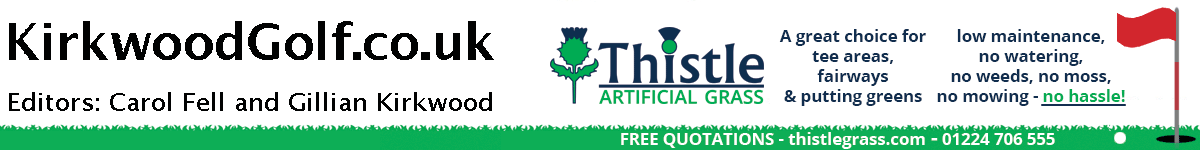KirkwoodGolf## Tuesday, March 29, 2016

Gemma Clews receives the trophy from Delamere Forest GC lady captain Annette Young.

Gemma Clews wins Comboy Scratc

Trophy for third year in a row

By COLIN FARQUHARSON
Gemma Clews, playing over her home course at Delamere Forest, Cheshire, has won the prestigious 36-hole Delamere Comboy Scratch Trophy for the third year in a row.
The +2 contender for a place in the GB and I Curtis Cup team for Dunlaoghaire GC, Ireland in June had rounds of 73 and 71 for 144 - three ahead of runner-up Hollie Muse (West Lancs).

Gemma Clews -2 73 + 71 = 144
Hollie Muse -3 73 + 74 = 147
Ebonie Lewis 2 74 + 76 = 150
GEORGIA PRICE 2 77 + 74 = 151
Bronwyn Davies 0 71 + 81 = 152
Olivia Lowe 1 76 + 78 = 154
Ashley CROFT 2 80 + 75 = 155
Lily May Humphreys 1 78 + 77 = 155
EMILY TOY -1 81 + 76 = 157
POPPY FINLAY -1 78 + 79 = 157
Angharad Evans JNR 3 77 + 80 = 157
Holly MORGAN -2 76 + 81 = 157
Rebecca Earl 1 75 + 82 = 157 157
JESSICA HALL 0 80 + 79 = 159
Alex Georgia Forrest 4 79 + 80 = 159
Melissa Wood 3 85 + 75 = 160
Natasha Slater -1 82 + 78 = 160
Louisa A Brunt 0 82 + 79 = 161
Emily Brennan 4 82 + 79 = 161
BRIONY BAYLES 5 86 + 76 = 162
SARAH COUSINS 2 84 + 78 = 162
Ainee O'Connor 3 81 + 81 = 162
Brandy Rook 3 81 + 82 = 163 163
Emily McBurney 5 79 + 84 = 163

Caley McGinty 5 82 + 83 = 165
Nicole Stewart 4 81 + 84 = 165
G ALLMAN 2 79 + 86 = 165
Fern Clark 4 84 + 82 = 166
Angharad Basnett 4 82 + 84 = 166
Jessica Murray 5 86 + 81 = 167
Alexandra Giles 5 83 + 84 = 167
Caroline Elizabe Berry 2 87 + 83 = 170
Nicola Slater 4 86 + 84 = 170
F. BOUGEN 3 85 + 85 = 170
KATRINA GILLUM 1 84 + 86 = 170
Lauren Drake 5 81 + 89 = 170
Billie-Jo Smith 1 88 + 83 = 171
Sophie FAULKNER 5 87 + 84 = 171
Nicola George 5 83 + 88 = 171 171
Lucy Jamieson (j) 3 87 + 85 = 172
Caitlin WHITEHEAD 2 83 + 89 = 172
Victoria Turner 6 82 + 90 = 172

Labels: